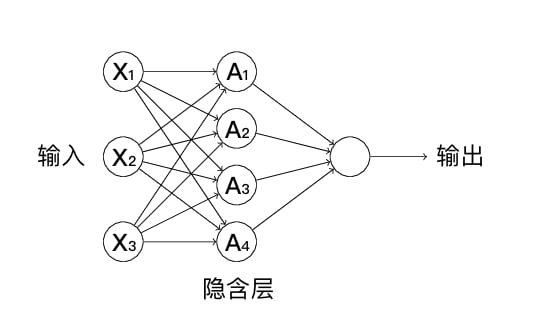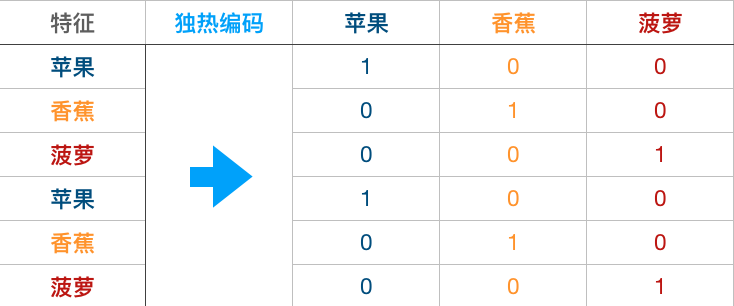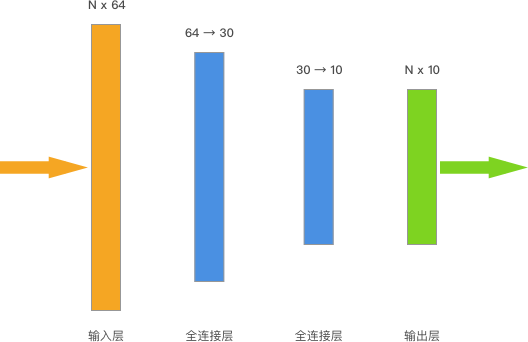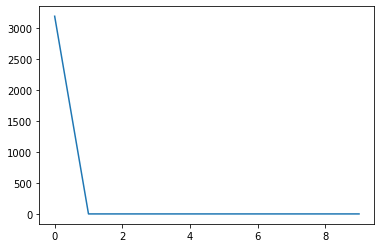﻿ 使用 TensorFlow 搭建多层神经网络 - Nemo

# 使用 TensorFlow 搭建多层神经网络### 使用 TensorFlow 搭建多层神经网络

#### 挑战介绍

• TensorFlow 原理及使用
• 多层神经网络

#### 内容### 实现全连接层

import tensorflow as tf

def fully_connected(input_layer, weights, biases):
&quot;&quot;&quot;
参数:
input_layer -- 输入层 Variable
weights -- 权重项 Variable
biases -- 截距项 Variable

返回:
output -- 输出层 Variable
&quot;&quot;&quot;
### 代码开始 ### (≈ 2 行代码)
layer = None
output = None
### 代码结束 ###
return output

import tensorflow as tf

def fully_connected(input_layer, weights, biases):
&quot;&quot;&quot;
参数:
input_layer -- 输入层 Variable
weights -- 权重项 Variable
biases -- 截距项 Variable

返回:
output -- 输出层 Variable
&quot;&quot;&quot;
### 代码开始 ### (≈ 2 行代码)
output = tf.nn.relu(layer)
### 代码结束 ###
return output

input_layer = tf.Variable(tf.random_normal([4, 4], stddev=10, seed=10))
weights = tf.Variable(tf.random_normal([4, 2], stddev=10, seed=10))
biases = tf.Variable(tf.random_normal(, stddev=10, seed=10))
fully_connected(input_layer, weights, biases)

&lt;tf.Tensor &#039;Relu:0&#039; shape=(4, 2) dtype=float32&gt;

### 代码开始 ### (≈ 4 行代码)

### 代码结束 ###

output

# 代码开始 ### (≈ 4 行代码)
init = tf.global_variables_initializer()
with tf.Session() as sess:
sess.run(init)
output = sess.run(fully_connected(input_layer, weights, biases))
### 代码结束 ###
output

array([[  0.     ,   0.     ],
[  0.     , 286.99103],
[371.80936,   0.     ],
[  0.     ,   0.     ]], dtype=float32)

### 数据预处理

import numpy as np
from sklearn import datasets

digits = datasets.load_digits()

images 8×8 矩阵，记录每张手写字符图像对应的像素灰度值
data 将 images 对应的 8×8 矩阵转换为行向量
target 记录 1797 张影像各自代表的数字

train_x = tf.Variable(digits.data, dtype=&quot;float32&quot;)
train_y = tf.one_hot(tf.Variable(digits.target), depth=10)### 搭建多层神经网络实现手写字符识别digits.target.shape, train_y.shape # 查看前后形状的变化


&quot;&quot;&quot;完成 2 个全连接层
&quot;&quot;&quot;

input_layer = train_x # 输入层

### 代码开始 ### (≈ 4 行代码)

# 全连接层 1
weight_1 = None
bias_1 = None
fc_layer_1 = None

# 全连接层 2
weight_2 = None
bias_2 = None
fc_layer_2 = None

### 代码结束 ###
output_layer = fc_layer_2 # 输出层


&quot;&quot;&quot;完成 2 个全连接层
&quot;&quot;&quot;

input_layer = train_x  # 输入层

# 代码开始 ### (≈ 4 行代码)

# 全连接层 1
weight_1 = tf.Variable(tf.ones([64, 30]))
bias_1 = tf.Variable(tf.ones())
fc_layer_1 = fully_connected(input_layer, weight_1, bias_1)

# 全连接层 2
weight_2 = tf.Variable(tf.ones([30, 10]))
bias_2 = tf.Variable(tf.ones())
fc_layer_2 = fully_connected(fc_layer_1, weight_2, bias_2)

### 代码结束 ###
output_layer = fc_layer_2  # 输出层


# 交叉熵损失函数，该步骤无需理解，后面实验详细介绍
loss = tf.reduce_mean(tf.nn.softmax_cross_entropy_with_logits_v2(labels=train_y, logits=output_layer))

# 定义损失函数和优化器
### 代码开始 ### (≈ 1～2 行代码)

train_step = None

### 代码结束 ###


# 交叉熵损失函数，该步骤无需理解，后面实验详细介绍
loss = tf.reduce_mean(tf.nn.softmax_cross_entropy_with_logits_v2(
labels=train_y, logits=output_layer))

# 定义损失函数和优化器
# 代码开始 ### (≈ 1～2 行代码)

### 代码结束 ###


from matplotlib import pyplot as plt
%matplotlib inline

### 代码开始 ### (≈ 8~10 行代码)

### 代码结束 ###


from matplotlib import pyplot as plt
%matplotlib inline

# 代码开始 ### (≈ 8~10 行代码)
loss_list = []

init = tf.global_variables_initializer()
with tf.Session() as sess:
sess.run(init)
for _ in range(10):
sess.run(train_step)
loss_list.append(sess.run(loss))

plt.plot(loss_list)
loss_list
### 代码结束 ###


[3191.4922,
2.3109665,
2.310335,
2.3097448,
2.3091934,
2.3086793,
2.3082006,
2.307755,
2.3073409,
2.3069558]Nemo版权所有丨如未注明，均为原创丨本网站采用BY-NC-SA协议进行授权，转载请注明转自：https://nemo.cool/239.html### Nemo

#### 文章作者2019-07-21